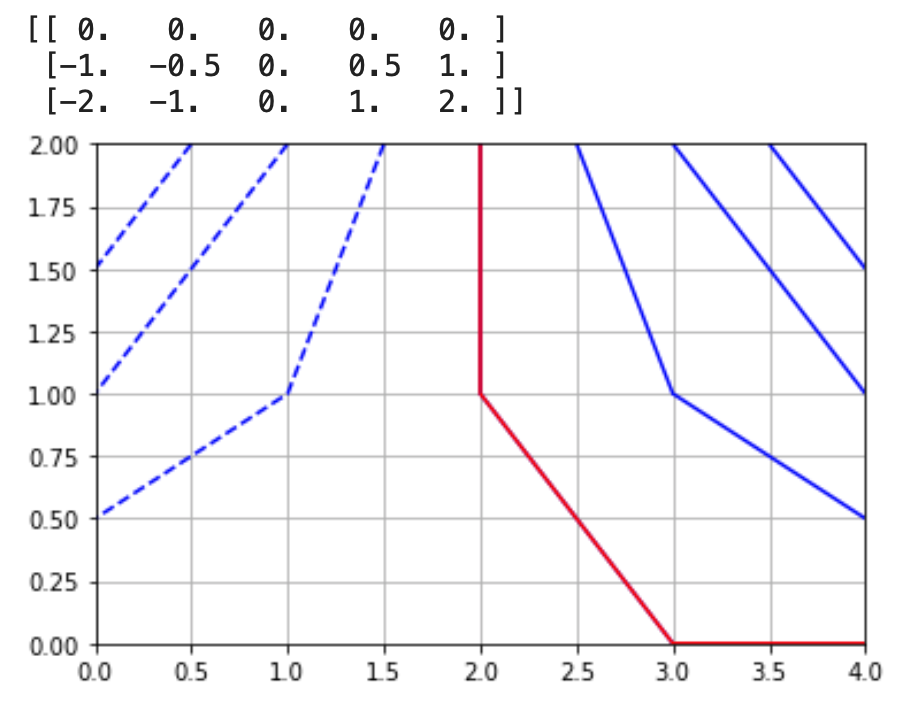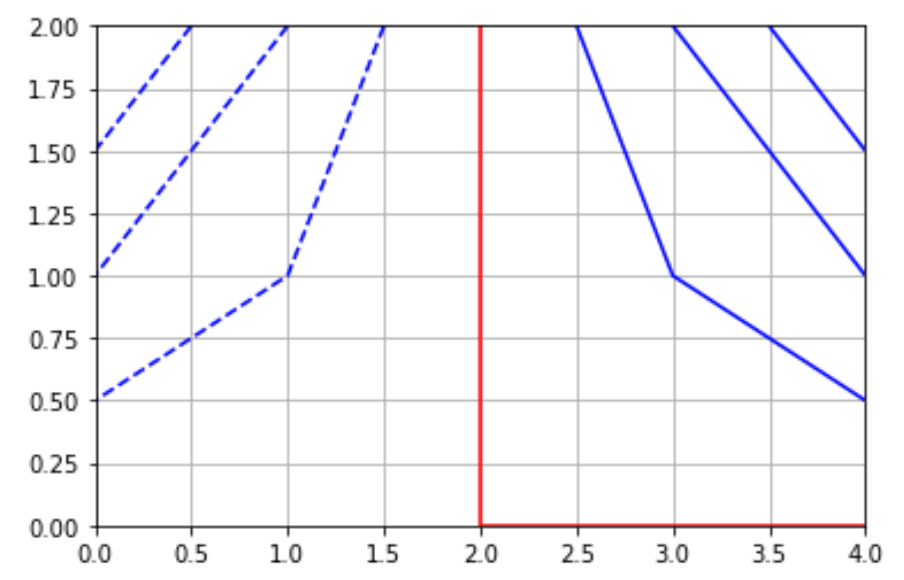# contour problem 90 degree angle

### Bug report

Bug summary

The contour routine seems to produce strange results when a contour line must make a 90 degree angle. The problem is reproduced by contouring a small array with 3 rows and 5 columns. The value of the array equals zero along row 0 and along column 2 (the middle column). Contouring the 0 value does not follow this column and the first row.

Code for reproduction

``````import numpy as np
import matplotlib.pyplot as plt
N = 5
h = np.empty((3, N))
h = 0
h = np.linspace(-1, 1, N)
h = np.linspace(-2, 2, N)
print(h)
plt.contour(h, [-1.5, -1, -0.5, 0, 0.5, 1, 1.5], colors='b')
plt.contour(h, , colors='r')
plt.grid()
``````

Actual outcomeExpected outcome

The red line, which represents the line for which `h=0` should have gone straight down to (2,0) and then make a 90 degree angle at the bottom of the figure. In fact, the entire bottom row of the figure equals 0.Matplotlib version

• Operating system: MacOS
• Matplotlib version 3.3.2
• Matplotlib backend MacOS
• Python version: 3.8 (standard Anaconda distribution)
• Jupyter version: Jupyter Lab 2.2.6
• Other libraries: numpy 1.19.2Full dicussion on github.com

## 1 possible answer(s) on “contour problem 90 degree angle”

1. Hi @mbakker7, you have posted a good clear example.

The short answer is that anyone using a dataset that contains a number of adjacent values which exactly match a contour level are likely to be disappointed with the results.

The full answer is much longer…

You are asking for a red contour line to be drawn at `z=0`. Looking at your problematic quadrilateral (`2 <= x <= 3`, `0 <= y <= 1`), 3 of the 4 corners are at `z=0`. How should a contour line at `z=0` be drawn here? There are a number of possible answers, all of which are ‘reasonable’ to some extent. Because 3 of the corners are at `z=0` it is reasonable to consider the whole triangle bounded by those points to be at `z=0`. Hence perhaps the contour line should expand from being its normal narrow (1 or so pixels wide) line to fill the whole triangle? It would be reasonable rendering of the data, but obviously you have requested a contour line so it shouldn’t be drawn any wider. You’d like it to be rendered as the left and bottom boundaries of that triangle, which is also reasonable. Equally reasonable is the rendered line which is the right-hand side of the triangle. It could be argued that those two line options are extreme and some sort of average position should be used, e.g. a line from the top to `x=2.5`. But that solution will annoy everybody! We cannot render all of these reasonable options, we have to make a decision to do one of them and apply it consistently.

The algorithm is primarily edge-based. It classifies each of the points in your grid as being above or below the contour level. If an edge of a quad has one end above and the other below the contour level, it knows that contour crosses that edge. But ‘above’ and ‘below’ the contour level are not sufficient, we have to consider ‘equals’ the contour level. So for ‘above’ we use `z > contour_level` and for below the logical opposite which is `z <= contourlevel`. Therefore the top-right point of your quad in question is classified as ‘above’, the other 3 are ‘below’. The algorithm determines the contour line passes through the top and right edges of the quad, and simply connects them. If we switched above to `z >= contour_level` then this quad will be OK but the quad to the left will be drawn this way instead! We can’t get rid of it, if you use data values and contour levels that match (to floating-point numerical precision) then the current algorithm with give one of the ‘reasonable’ results, which unfortunately isn’t the one you want.

It would be possible to write a new algorithm that better considers the internals of each quad and does something different to just connecting the edges that the contour crosses. The problem then is finding someone willing to do this work and motivating them to do it.

But the takeaway message here is not to try to plot narrow contour lines over a region that matches the contour level because you are likely to be disappointed with the results.